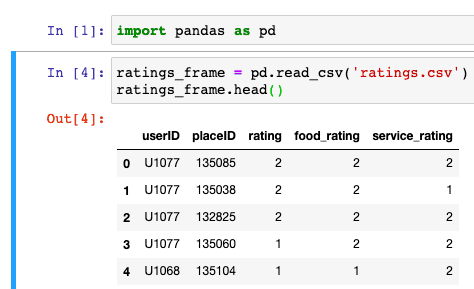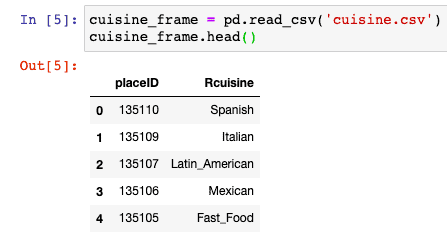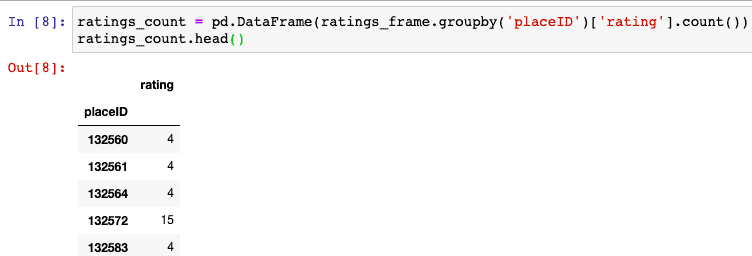# Pandas DataFrame groupby: The Complete Guide

Pandas DataFrame groupby() method is used to split data of a particular dataset into groups based on criteria. The groupby() function splits the data on any of the axes.

## Pandas groupby

Pandas groupby() is a built-in library method used to group data objects into Series (columns) or DataFrames (a group of Series) based on particular indicators.

The groupby in Python makes the management of datasets easier since you can put related records into groups.

Pandas DataFrame groupby() function involves the splitting of objects, applying some function, and then combining the results. It is usually done on the last group of data to cluster the data and take out meaningful insights from the data.

Let’s take a look at the df.groupby() method itself.

```import pandas as pd

dataset = {
'Name': ['Rohit', 'Arun', 'Sohit', 'Arun', 'Shubh'],
'Roll no': ['01', '02', '03', '04', '05'],
'maths': ['93', '63', '74', '94', '83'],
'science': ['88', '55', '66', '94', '35'],
'english': ['93', '74', '84', '92', '87']}

df = pd.DataFrame(dataset)
by_name = df.groupby(['Name'])
print(by_name)
```

#### Output

`<pandas.core.groupby.generic.DataFrameGroupBy object at 0x10e965250>`

In the output, what is that DataFrameGroupBy thing? It is a .__str__() that doesn’t give you much information into what it is or how it works. The reason that the DataFrameGroupBy object can be challenging to wrap your head around is that it’s lazy. It doesn’t do any operations to produce a useful result until you say so.

One term that’s frequently used alongside the .groupby() method is split-apply-combine. This refers to the chain of the following three steps:

1. Split a DataFrame into groups.
2. Apply some operations to each of those smaller DataFrames.
3. Combine the results.

It can be challenging to inspect df.groupby(“Name”) because it does virtually nothing of these things until you do something with a resulting object. Again, the Pandas GroupBy object is lazy. It delays almost any part of the split-apply-combine process until you call a method on it.

So, how can you separate the split, apply, and combine stages if you can’t see any of them happening in isolation? One useful way to inspect the Pandas GroupBy object and see the splitting in action is to iterate through it. This is implemented in DataFrameGroupBy.__iter__() and outputs an iterator of (group, DataFrame) pairs for DataFrames.

```import pandas as pd

dataset = {
'Name': ['Rohit', 'Arun', 'Sohit', 'Arun', 'Shubh'],
'Roll no': ['01', '02', '03', '04', '05'],
'maths': ['93', '63', '74', '94', '83'],
'science': ['88', '55'a '66', '94', '35'],
'english': ['93', '74', '84', '92', '87']}

df = pd.DataFrame(dataset)
by_name = df.groupby(['Name'])

for Name, maths in by_name:
print(f"First 2 entries for {Name!r}")
print("------------------------")
```

#### Output

```First 2 entries for 'Arun'
------------------------
Name Roll no maths science english
1  Arun      02    63      55      74
3  Arun      04    94      94      92

First 2 entries for 'Rohit'
------------------------
Name Roll no maths science english
0  Rohit      01    93      88      93

First 2 entries for 'Shubh'
------------------------
Name Roll no maths science english
4  Shubh      05    83      35      87

First 2 entries for 'Sohit'
------------------------
Name Roll no maths science english
2  Sohit      03    74      66      84```

If you’re working on the difficult aggregation problem, then iterating over a Pandas GroupBy object can be a considerable way to visualize a split part of split-apply-combine.

There are very few other methods and properties that let you look into the individual groups and their splits. The .groups attribute will give you the dictionary of {group Name: group label} pairs.

Now, let’s head back to its syntax.

### Syntax

```DataFrame.groupby(by=None, axis=0, level=None, as_index=True, sort=True, group_keys=True, squeeze=False, **kwargs)
```

### Parameters

The groupby() function contains 7 parameters.

1. by: It is used to determine the groups for the groupby() function. Its default value is none. It is the mapping function.
2. axis: It takes integer values; by default, it is 0.
3. level: If the axis is a MultiIndex that is hierarchical, the grouping is done by a particular level or multiple levels.
4. as_index: It is of the Boolean data type. For aggregated output, we return the object with group labels as the index. It is only relevant for DataFrame input.
5. sort: Sort group keys. We get better performance by turning this off.
6. group_keys: It is also of Boolean data type and has the value true by default. When calling apply, add group keys to the index to identify pieces.
7. Squeeze: It is also of the Boolean data type by default, it is False. It reduces the dimensionality of the return type if possible. Otherwise, it returns a consistent type.

### Return Value

The groupby() function returns a groupby object that contains information about the different groups.

### Example program on Pandas DataFrame groupby()

Write a program to show the working of the groupby() method in Python.

```import pandas as pd

dataset = {
'Name': ['Rohit', 'Mohit', 'Sohit', 'Arun', 'Shubh'],
'Roll no': ['01', '02', '03', '04', '05'],
'Maths': ['93', '63', '74', '94', '83'],
'Science': ['88', '55', '66', '94', '35'],
'English': ['93', '74', '84', '92', '87']
}

df = pd.DataFrame(dataset)
group = df.groupby('Roll no')
print(group.first())```

#### Output

```Name Maths Science English
Roll no
01       Rohit    93      88      93
02       Mohit    63      55      74
03       Sohit    74      66      84
04        Arun    94      94      92
05       Shubh    83      35      87```

In the above example, we can see that there is a dataset that contains data of the student, and we have grouped that data based on Roll no.

### Write a program to group data by multiple columns.

```import pandas as pd

dataset = {
'Name': ['Rohit', 'Arun', 'Sohit', 'Arun', 'Shubh'],
'Roll no': ['01', '02', '03', '04', '05'],
'maths': ['93', '63', '74', '94', '83'],
'science': ['88', '55', '66', '94', '35'],
'english': ['93', '74', '84', '92', '87']}

df = pd.DataFrame(dataset)
group = df.groupby(['Name', 'Roll no'])
print(group.first())
```

#### Output

```maths science english
Name  Roll no
Arun  02         63      55      74
04         94      94      92
Rohit 01         93      88      93
Shubh 05         83      35      87
Sohit 03         74      66      84```

In the above example, we can see that we have done grouping on multiple columns, i.e., Name and Roll no.  Here we can see that we have 2 Arun’s in the class with different roll numbers; hence grouping is done accordingly.

## Learn Pandas df.groupby() on Jupyter Notebook

Jupyter Notebook by Anaconda is an essential tool for working on Machine Learning and Data Science. We will create a DataFrame from external CSV data and then use the groupby method to fetch the data based on different requirements.

Now, we will use the following two datasets.

1. ratings.csv
2. cuisine.csv

So, we will create two DataFrames from these CSV data.

Let’s import Pandas and create a first DataFrame using the Pandas read_csv() method.

`import pandas as pd`

Now, create ratings _frame DataFrame.

```ratings_frame = pd.read_csv('ratings.csv')

Run the cell, and you will get the following output.The next step is to create a cuisine_frame DataFrame.

```cuisine_frame = pd.read_csv('cuisine.csv')From the DataFrame outputs, you can see that both DataFrames are connected via placeID.

If you have learned SQL, you can recall the concept of Primary Key and Foreign Key.

So, Foreign Key in ratings _frame is placeID.

Now, let’s count the ratings of each first five placeIDs.

The ratings_frame has all the data we need. So let’s use the groupby() function to count the rating placeID wise.

```ratings_count = pd.DataFrame(ratings_frame.groupby('placeID')['rating'].count())You call .groupby() method and pass the name of the column you want to group on, which is “placeID”. Then, you use [“rating”] to define the columns on which you have to operate the actual aggregation.

You can pass a lot more than just a single column name to .groupby() method as the first argument. You can also cite any of the following:

1. A list of multiple column names
2. The dict or Pandas Series
3. Numpy array or Pandas Index, or an array-like iterable of these

You can see that we have fetched the count of ratings for the first five placeIDs.

The analogous SQL query would look like the following.

```SELECT placeID, count(rating)
FROM df
GROUP BY placeID;```

## Pandas groupby vs. SQL groupby

Most often, the aggregation capacity is compared to the GROUP BY clause in SQL. However, there are differences between how SQL GROUP BY and groupby() in DataFrame operates.

Unlike SQL, the Pandas groupby() method does not have a concept of ordinal position references. Thus, you will need to reference the grouping keys by Name explicitly.

Pandas DataFrames are versatile in terms of their capacity to manipulate, reshape, and munge data. One of the prominent features of the DataFrame is its capability to aggregate data.

## Pandas GroupBy object methods

1. Aggregation methods “smush” many data points into an aggregated statistic about those data points. For example, you can take a sum, mean, or median of 10 numbers, where a result is just a single number.
2. Filter methods come back to you with the subset of the original DataFrame. This most commonly means using the .filter() method to drop entire groups based on some provisional statistic about that group and its sub-table. It also makes sense to include under that definition several methods that exclude specific rows from each group.
3. Transformation methods return the DataFrame with the same shape and indices as an original, but with different values. With both aggregation and filter methods, a resulting DataFrame will commonly be smaller in size than the input DataFrame. This is not true of the transformation, which transforms individual values themselves but retains the shape of the original DataFrame.
4. Meta methods are less concerned with an original object on which you called .groupby(), and more focused on giving you high-level information such as the number of groups and indices of those groups.
5. Plotting methods mimic the API of plotting for a Pandas Series or DataFrame, but typically break the output into multiple subplots.

## Conclusion

The groupby is a method in the Pandas library that groups data according to different sets of variables. Finally, the Pandas DataFrame groupby() example is over.